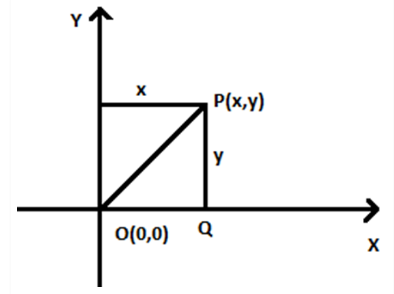# Distance Between Two Points Formula

Distance between two points formula uses the coordinates of two points in space. In coordinate geometry, the distance formula is used to find the distance between two points present in a two-dimensional or three-dimensional space.

In case of a two-dimensional plane, the two points lie along x-axis and y-axis. The distance of a point from the y-axis is known as its x-coordinate or abscissa. The distance of a point from the x-axis is known as its y-coordinate, or ordinate. The coordinates of a point on the x-axis and y-axis are of the form (x, 0) and (0, y), respectively.## Distance Formula for Two Points

As discussed, the distance formula is used to find the distance between any two points, when we already know the coordinates. The points could be present alone in x-axis or y-axis or in both the axes.

Let us consider, there are two points say A and B in an XY plane. The coordinates of point A are (x1,y1) and of B are (x2,y2). Then the formula to find the distance between two points PQ is given by:

$$AB = \sqrt{[x_{2}-x_{1}]^{2}+[y_{2}-y_{1}]^{2}}$$

### How to find the distance between two points on a coordinate plane?

Suppose there are two points in a plane P (2,3) and Q(-2,0). Now, how can we determine the distance between P and Q. Thus, we will use the above equation here.

Here, x1 = 2, x2 = -2, y1=3 and y2 = 0.

Now, putting these values in the distance formula, we get;

$$PQ = \sqrt{[-2-2]^{2}+[0-3]^{2}}\\ PQ = \sqrt{-4^{2}+-3^{2}}$$

PQ = √(16+9) = √25 = 5 unit.

Hence, we have found the distance between points P and Q.

### Distance Between Two Points Formula in 3D Space

If we have to find the distance between the points in a three-dimensional space, then we consider here an extra coordinate which is present in the z-axis.

Let us consider two points A(x1,y1,z1) and B(x2,y2,z2) in 3d space. Then, the distance formula for these two points is given by;

$$AB = \sqrt{[x_{2}-x_{1}]^{2}+[y_{2}-y_{1}]^{2}+[z_{2}-z_{1}]^{2}}$$

$$AB = \sqrt{x^{2}+y^{2}+z^{2}}$$